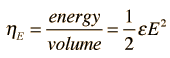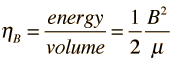# Energy in Electric and Magnetic Fields

Both electric fields and magnetic fields store energy. For the electric field the energy density isShow

This energy density can be used to calculate the energy stored in a capacitor.

For the magnetic field the energy density isShow

which is used to calculate the energy stored in an inductor.

For electromagnetic waves, both the electric and magnetic fields play a role in the transport of energy. This power is expressed in terms of the Poynting vector.

Index

Voltage concepts

Electric field concepts

 HyperPhysics***** Electricity and Magnetism R Nave
Go Back